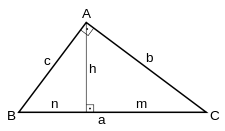# HypotenuseA right-angled triangle and its hypotenuse.

In geometry, a hypotenuse (rarely: hypothenuse) is the longest side of a right-angled triangle, the side opposite of the right angle. The length of the hypotenuse of a right triangle can be found using the Pythagorean theorem, which states that the square of the length of the hypotenuse equals the sum of the squares of the lengths of the other two sides. For example, if one of the other sides has a length of 3 (when squared, 9) and the other has a length of 4 (when squared, 16), then their squares add up to 25. The length of the hypotenuse is the square root of 25, that is, 5.

## Etymology

The word hypotenuse means essentially "length under", and derives from Latin hypotēnūsa, a transliteration of Ancient Greek hypoteínousa (pleurā́ or grammḗ), the feminine present participle of hypoteínō, a combination of hypó ("under") and teínō ("I stretch" or "length"). The word ὑποτείνουσα was used for the hypotenuse of a triangle by Plato in the Timaeus (dialogue) 54d and by many other ancient authors.

A folk etymology says that tenuse means "side", so hypotenuse means a support like a prop or buttress, but this is inaccurate.

## Calculating the hypotenuseThe length of the hypotenuse is calculated using the square root function implied by the Pythagorean theorem. Using the common notation that the length of the two legs of the triangle (the sides perpendicular to each other) are a and b and that of the hypotenuse is c, we haveThe Pythagorean theorem, and hence this length, can also be derived from the law of cosines by observing that the angle opposite the hypotenuse is 90° and noting that its cosine is 0:Many computer languages support the ISO C standard function hypot(x,y), which returns the value above. The function is designed not to fail where the straightforward calculation might overflow or underflow and can be slightly more accurate.

Some scientific calculators provide a function to convert from rectangular coordinates to polar coordinates. This gives both the length of the hypotenuse and the angle the hypotenuse makes with the base line (c1 above) at the same time when given x and y. The angle returned will normally be that given by atan2(y,x).

## PropertiesIn the figure, a is the hypotenuse and b and c are the catheti. The orthographic projection of b is m, and of c is n.
• The length of the hypotenuse equals the sum of the lengths of the orthographic projections of both catheti. And
• The square of the length of a cathetus equals the product of the lengths of its orthographic projection on the hypotenuse times the length of this.
b² = a · m
c² = a · n
• Also, the length of a cathetus b is the proportional mean between the lengths of its projection m and the hypotenuse a.
a/b = b/m
a/c = c/n

## Trigonometric ratios

By means of trigonometric ratios, one can obtain the value of two acute angles,and, of the right triangle.

Given the length of the hypotenuseand of a cathetus, the ratio is:The trigonometric inverse function is:in whichis the angle opposite the cathetus.

The adjacent angle of the catheti, will be= 90° –One may also obtain the value of the angleby the equation:in whichis the other cathetus.